Arrays in C# .Net
Ratings:
(4)
Views:147## Arrays

Arrays are of type “reference” That is even if an Array is not initialized still it holds some default values.

int a, b, c ; C = a + b; STACK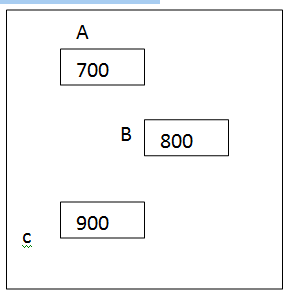Array  X(5)       x=5000 HEAP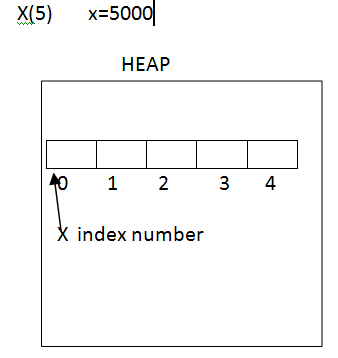The array is a collection of homogeneous data type Arrays shares the same name with different index values Always index starts from ‘0’ Arrays will be stored in continuous memory Arrays are faster in accessing All the arrays are instance of the system. Array class

These core tutorials will help you to learn the fundamentals of .NET. For an in-depth understanding and practical experience, explore Online ".NET Training"

↓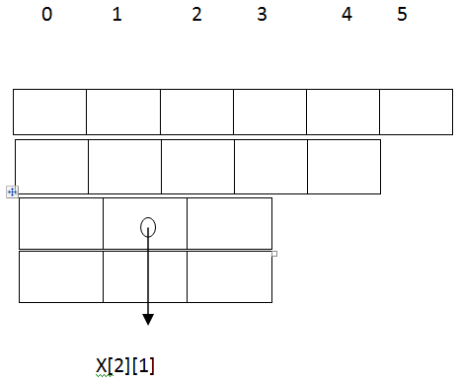C# supports three types of Arrays One-dimensional arrays Multidimensional arrays Jagged Arrays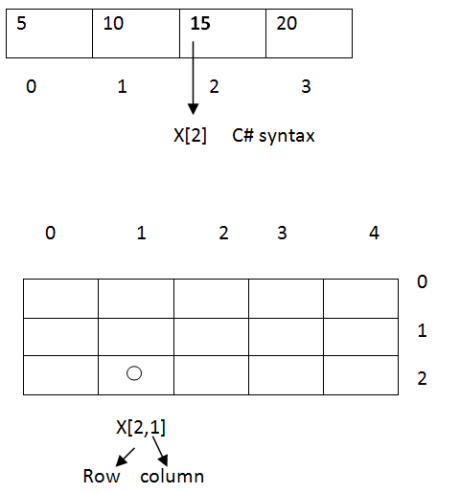## Working with a one-dimensional array

Syntax Data type []  arr_name  = new data type [size];

Example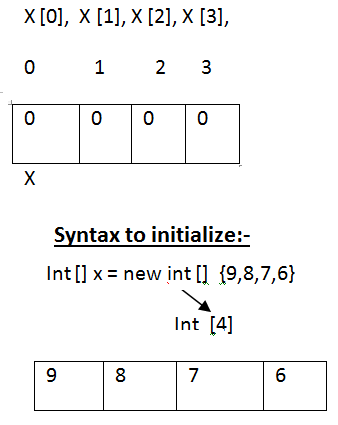While initializing an array, size is not recommended

Example

A program to print array default rules:-

Open window forms application project Place a button Code for button 1

``````click

{

Int [] x = new int ;

For  (int  I =0 ; i< arg.length ; I ++);

Message Box. Show ( x [i]+ “”);

}

``````

Output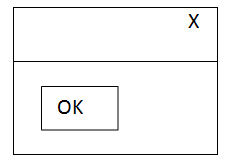Observations

The integral Array default value is Zero. Floating Array default value is Zero.

char Array default value is nothing. Bool Array default value is false.

Date time Array default value is 1/1/0001 12:00:00 AM.

### Example

A program to create or get the spelling of a given number     (1…………………..999) 123…………..one hundred twenty-three Open windows forms application project Place two text boxes and a button``````Code for button1

click

{

Int n = int. parse (text box 1.text);

Int I = 0;

String res = “”;

String [] ones  =  new string []

{ “one”, “two”, “three”, “four” “five”, “six” ………………,” nineteen”};

String [] Tens = new string []

{“ten”, “twenty”, “ninety”};

N = 426 N = 426/ 100  -à 10/3 = 3

If (n > 99 && n < 1000)

{

I = n / 100; Res = ones [I - 1] + “hundred”; N = n % 100;

}

If (n > 19 && n < 1000)

{

I = n / 100; Res = ones [I - 1] + “hundred”;

N = n % 100;

}

If (n > 0&& n < 20)

Res =res +  ones [I - 1] ;

Text Box 2.text = res;

F5

}

``````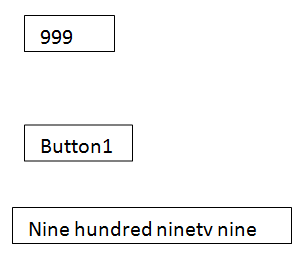## Working with Multi Dimensional Arrays

Arranging a set of elements in rows and columns is called  “Multi-Dimensional Array”.

In a Multi-Dimensional Array, every row must contain the same number of elements. Multi-Dimensional Array size will be represented with no. of rows X  no. of columns If the array name is ‘X’ then x. length return a total number of elements.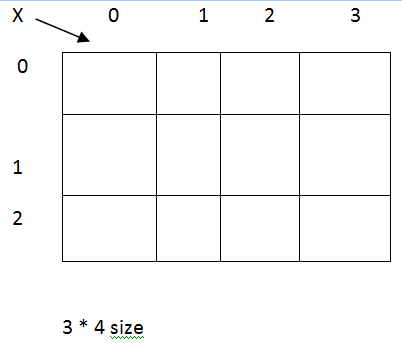get length (0) returns no. of rows get length (1) returns no. of columns syntax to declare multi – d array I0 : int [] x = new int [s]; S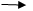20 : int [,] x = new int [s,s]; 30 : int [,,] x = new int [s,s,s];

Note VB supports up to 32 dimensions where as no limit in C #.NET Syntax to initialize 2 D Array   Int [,] x = new int [,] { {5,6,7,8} {9,8,7,6} {5,5,5,5} }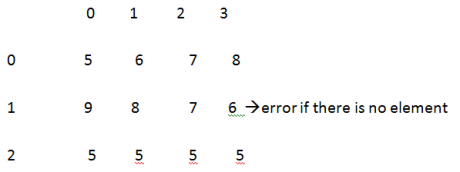Example with multi dimension Array

Open windows forms application project Place a button Code for button 1

``````click

{

String  s = “”;

Int [,]  x = new int [,];

{ {5, 6, 7, 8}, {9, 8, 7, 6}, {5, 5, 5, 5} };

r=0                  c = 0 Rr=1                    c =1 c =2 c =3 message  box. Show (“Total :” + x. length) ;

message box .show  (“Rows : + x. get length  (0)”) + “ cols :”  + x. get length  (1) );

For (int  r = 0; r < x. get length  (0);r ++)

{

For (int  c = 0; c< x. get length  (0);c ++)

{

S = S + X [r,c] + “” ;

}

S= s + “/n”

}

Message Box. Show (s);

``````

### Jagged Arrays

ABC(Company) Members are working Employees3*6values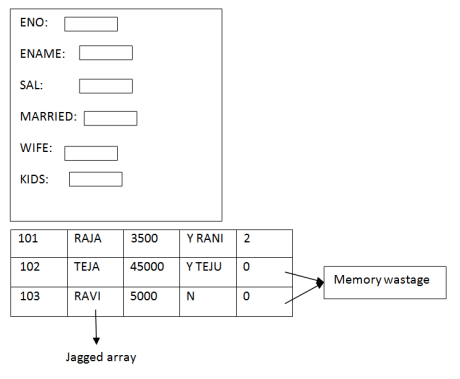A jagged array is a collection of rows where every row may contain a different number of elements. Jagged arrays save memory. Jagged arrays are faster in accessing. Jagged arrays are also called as “Dynamic array” (run time array) Jagged array is also called an array of arrays.

Syntax Int []  [] x = new int  []; X  = new int [] {4,2,3,5,6,7} X  = new int [] {3,8,4,2,4}

Example Open windows forms application project Place a button Code for button 1

``````click

{

Int [] []   x = new int [];

X  = new int [] {5,3,4,6,7,7};

X  = new int ;

X  = new int []{2,3};

String  S = “”;

For  (int r  = 0;r < x.get length (0);r ++)

{

For  (int c  = 0;r < x.get length (0);c++)

{

S= s + x[ r][c] +””;

}

S =s + “/n”

}

Message Box. Show (s);  ``````